# 1705 The Seat Numbers from Jimmy Fallon’s Twelve Days of Christmas Sweaters

### Today’s Puzzle:

Jimmy Fallon’s Twelve Days of Christmas Sweaters tradition has become something I look forward to each December. The sweaters are one-of-a-kind masterpieces. I love when the sweaters are revealed. Jimmy reaches into a bright red Christmas stocking and randomly pulls out a number, the seat number of the winner of the sweater. Miraculously,  the winner of each sweater looks fabulous in it, no matter how big or small the winner is. I love this tradition, the sweaters, the winners modeling the sweaters, but I also love hearing the seat numbers. Each seat number has something special about it. (Just because it is a number!) By day three, I knew I wanted to blog about the numbers this year. The seat numbers were 295, 257, 314, 270, 419, 126, 256, 417, 433, 242, 232, and 120. I immediately knew something special about several of the numbers, but some of them I had to research. Can you figure out what is so special about each one?

Three of the seat numbers were primes. Which three?

One of those primes is both the fourth Fermat prime and the second-largest known Fermat prime. Which prime number is that?

Two of the numbers were palindromes (numbers that read the same forward and backward). Which two?

One of the seat numbers is equal to 1 × 2 × 3 × 4 × 5. Mathematicians write that as 5! Which seat number is equal to 5!?

One of the numbers is 10π rounded. Which one?

### How Do Some of the Seat Numbers Shape Up?

Two of the numbers were decagonal numbers. Which two?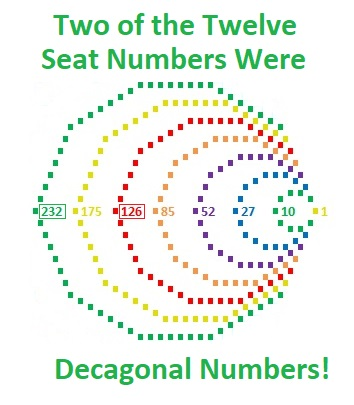126 is not only a decagonal number, but it is also a pyramid formed by stacking the first six pentagonal numbers on top of each other.
1 + 5 + 12 + 22 + 35 + 51 = 126.120 comes in THREE shapes.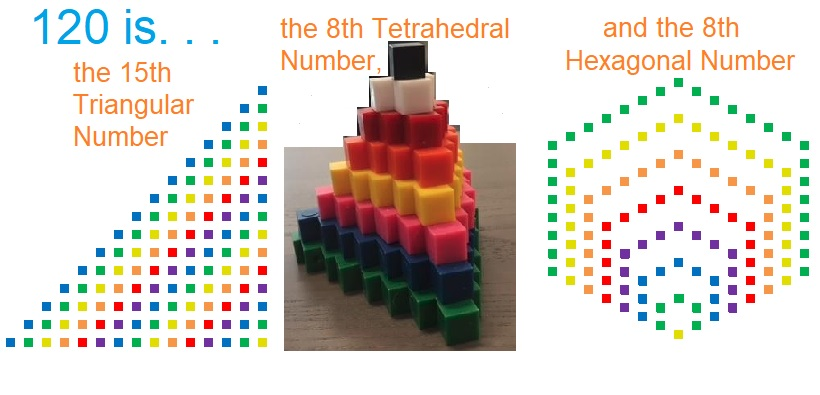One of the seat numbers is a star: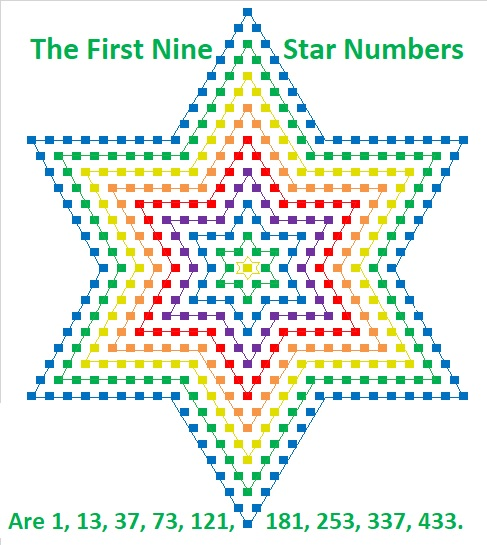### Something Special About Each Seat Number: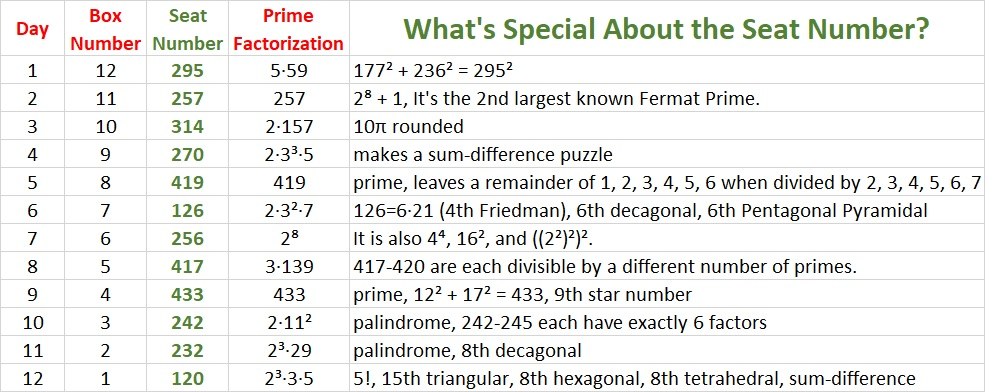I’ll explain some of these reasons below.

### Three of the Numbers Were the First Numbers to do Something Special:

242 is the smallest number whose square root can be simplified that is followed by three other numbers whose square root can also be simplified. Also, all four numbers have exactly six factors. Numbers with exactly six factors always have simplifiable square roots.

• 242 = 2·11²; its six factors are 1, 2, 11, 22, 121, 242.
• 243 = 3⁵; its six factors are 1, 3, 9, 27, 81, 243.
• 244 = 2²·61; its six factors are 1, 2, 4, 61, 122, 244.
• 245 = 7²·5; its six factors are 1, 5, 7, 35, 49, 245.417 is the smallest number that is the first of four consecutive integers that are divisible by a different number of primes.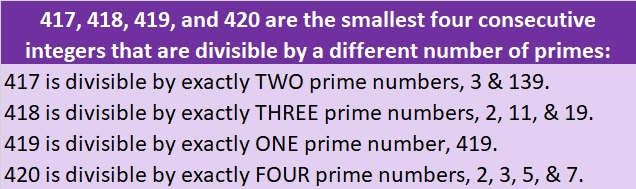419 is one less than 420, the smallest number divisible by 1, 2, 3, 4, 5, 6, and 7. As a consequence of that, 419 is the smallest number that leaves a remainder of 1, when it is divided by 2, a remainder of 2, when it is divided by 3, a remainder of 3, when it is divided by 4, a remainder of 4, when it is divided by 5, a remainder of 5, when it is divided by 6, and a remainder of 6, when it is divided by 7. This next graphic is a different way to make the same point.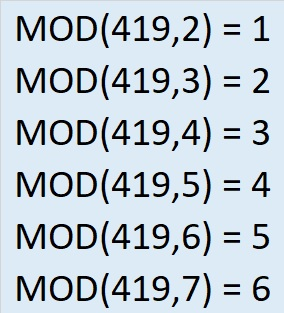### What Do I Mean by Sum-Difference?

Two of the seat numbers have factor pairs that make sum-difference: the numbers in one of its factor pairs add up to a particular number and the numbers in a different factor pair subtract to the same number. Coincidentally, both of the seat numbers are related to 30, another number that makes sum-difference.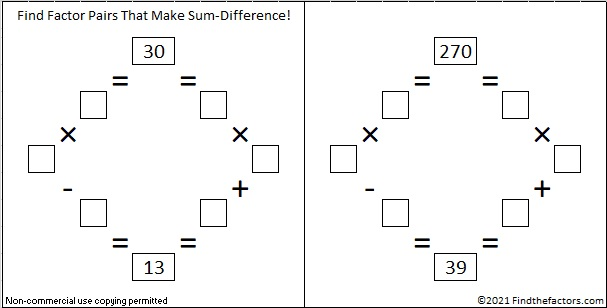I’ve so enjoyed discovering what made all those seat numbers special, and I hope that you have enjoyed reading about them as well!

Since this is my 1705th post, I’ll write a little about that number, as well.

### Factors of 1705:

• 1705 is a composite number.
• Prime factorization: 1705 = 5 × 11 × 31.
• 1705 has no exponents greater than 1 in its prime factorization, so √1705 cannot be simplified.
• The exponents in the prime factorization are 1, 1, and 1. Adding one to each exponent and multiplying we get (1 + 1)(1 + 1)(1 + 1) = 2 × 2 × 2 = 8. Therefore 1705 has exactly 8 factors.
• The factors of 1705 are outlined with their factor pair partners in the graphic below.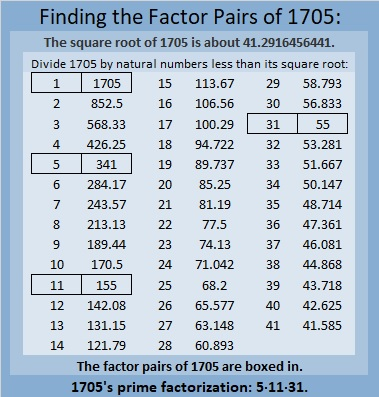### More About the Number 1705:

1705 is the hypotenuse of a Pythagorean triple:
1023 1364 1705, which is (3-4-5) times 341.

# 433 The Whole Is Greater Than the Sum of Its Parts

Today I practiced a song with a choir comprised of nine smaller choirs. Every time before I had only practiced the song with our smaller choir. As I listened to all the voices in this big choir while we sang in harmony, I thought, “A big choir sounds SO good. The whole is greater than the sum of its parts!”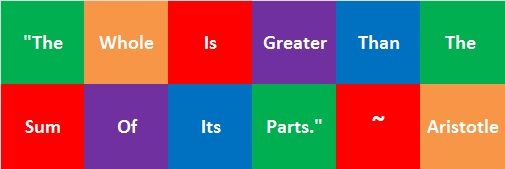Here is a professional recording of the beautiful song we are singing. The music in this youtube video is directed by the composer, Rob Gardner. It features the London Symphony Orchestra at Air Studios, London and the Spire Choir at Camelback Baptist Church in Paradise Valley, Arizona.

The number 433 is also greater than the sum of its parts.

12² + 17² = 433

A Chinese checker board looks like a star and has 121 holes so 121 is called a star number. The first star number is 1, followed by 13, 37, 73, and 121. The ninth star number is 433.

433 is one more than twelve times the eighth triangular number. Star numbers and triangular numbers are related like that.

145-408-433 is a primitive Pythagorean triple.

Here is the factoring information for the number 433:

• 433 is a prime number. 431 and 433 are twin primes.
• Prime factorization: 433 is prime.
• The exponent of prime number 433 is 1. Adding 1 to that exponent we get (1 + 1) = 2. Therefore 433 has exactly 2 factors.
• Factors of 433: 1, 433
• Factor pairs: 433 = 1 x 433
• 433 has no square factors that allow its square root to be simplified. √433 ≈ 20.8087How do we know that 433 is a prime number? If 433 were not a prime number, then it would be divisible by at least one prime number less than or equal to √433 ≈ 20.8087. Since 433 cannot be divided evenly by 2, 3, 5, 7, 11, 13, 17, or 19, we know that 433 is a prime number.